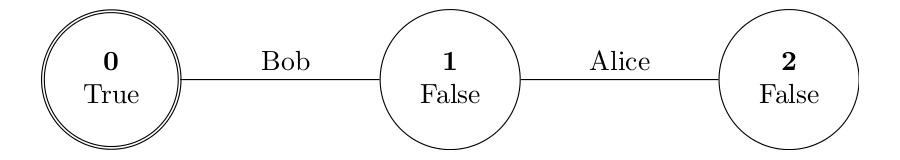KripkeVis

2014-04-22

To use this library you should provide three functions which take states, agents, valuations and models as inputs and return strings as output. If your data types are nothing special you can just the standard show function.
The module's functions take these three functions, some additional string and a model as input.

The module works best under Debian Linux and you should have Graphviz, pdfLaTeX and Okular installed. If not, the system() calls should be changed appropriately.

Also note that the directories "tmp/" and "tex/" should exist where you run it.

Try Online

You can see the module in action at Epistemic Logic Online.

Usage Example

The following lines are an example of how to use the module.
The result looks like this:module VISEXAMPLE where
import KRIPKEVIS

type Agent = Integer
alice, bob :: Agent
alice = 0
bob = 1

data MyModel = Mo [Int] [(Agent,[[Int]])] [(Int,Bool)] Int

exampleModel = Mo
[0,1,2]
[(bob,[[0,1],]),(alice,[,[1,2]])]
[(2,False),(0,True),(1,False)]
0

showAgent 0 = "Alice"
showAgent 1 = "Bob"

myDispModel :: MyModel -> IO String
myDispModel (Mo states rel val cur)
= dispModel show showAgent show "" (VisModel states rel val cur)

main :: IO ()
main = do
result <- myDispModel exampleModel
putStrLn result

Source Code

-- KRIPKEVIS.hs, version from 2014-04-22 by Malvin Gattinger

module KRIPKEVIS where
import Data.List
import System.IO
import System.Process

begintab  = "\\\\begin{tabular}{c}"
endtab    = "\\\\end{tabular}"
newline   = " \\\\\\\\[0pt] "

type PartitionOf a = [[a]]

data VisModel a b c = VisModel [a] [(b,PartitionOf a)] [(a,c)] a

stringModel :: Ord a => Eq a => Eq b => Show a => Show b =>
(a -> String) -> (b -> String) -> (c -> String) -> String
-> VisModel a b c
-> String
stringModel showState showAgents showVal information model =
"graph G { \n\
\  node [shape=doublecircle,label=\""
++ labelof cur ++ "\"] w" ++ showState cur ++ ";\n"
++ "  edge [lblstyle=auto]; \n"
++ concat [ ndlinefor s | s <- otherstates ]
++ "  rankdir=LR; \n size=\"8,5\" \n"
++ concat [   "  w" ++ show x ++" -- w"++ show y
++"[ label = \""++ showAgents a ++"\" ]; \n"
| (a,x,y) <- edges  ]
++ "  label = \""++information++"\"; \n "
++ "  fontsize=18; \n"
++ "} \n"
where
(VisModel states rel val cur) = model
labelof s = begintab ++ "\\\\textbf{" ++ showState s ++ "}" ++ newline ++ showVal (visApply val s) ++ endtab
ndlinefor s = "  node [shape=circle,label=\""
++ labelof s ++ "\"] w" ++ show s ++ ";\n"
otherstates = delete cur states
edges = nub $concat$ concat $concat [ [ [ if (x < y) then [(a,x,y)] else [] | x <- part, y <- part ] | part <- (visApply rel a) ] | a <- (agents model) ] agents (VisModel _ rel _ _) = map fst rel dotModel :: Ord a => Eq a => Eq b => Show a => Show b => (a -> String) -> (b -> String) -> (c -> String) -> String -> VisModel a b c -> String -> IO String dotModel showState showAgents showVal info model filename = let gstring = stringModel showState showAgents showVal info model in do newFile <- openFile (filename) WriteMode hPutStrLn newFile gstring hClose newFile return ("The model was DOT'd to "++filename) texModel :: Ord a => Eq a => Eq b => Show a => Show b => (a -> String) -> (b -> String) -> (c -> String) -> String -> VisModel a b c -> String -> IO String texModel showState showAgents showVal info model filename = let gstring = stringModel showState showAgents showVal info model in do forget <- dotModel showState showAgents showVal info model ("tex/"++filename++".dot") putStrLn forget pid <- system ("cd tex/; dot2tex --figonly -ftikz -traw -p --autosize --tikzedgelabels -w --usepdflatex "++filename++".dot > "++filename++".tex;" ) return ("Model was TeX'd into the file tex/" ++ filename ++ ".tex") dispModel :: Ord a => Eq a => Eq b => Show a => Show b => (a -> String) -> (b -> String) -> (c -> String) -> String -> VisModel a b c -> IO String dispModel showState showAgents showVal info model = let gstring = stringModel showState showAgents showVal info model in do forget <- dotModel showState showAgents showVal info model "tmp/temp.dot" putStrLn forget pid <- system ("cd tmp/; dot2tex -ftikz -traw -p --autosize --tikzedgelabels -w --usepdflatex temp.dot > temp.tex; pdflatex -interaction=nonstopmode temp.tex > temp.pdlatex.log; okular temp.pdf;" ) return ("Model was TeX'd and shown.") -- A helper function to apply relations: visApply :: Show a => Eq a => [(a,b)] -> a -> b visApply rel left = if (elem left ( map fst rel )) then snd$ head \$ filter (\(a,b) -> a==left) rel
else
error ("Applying of a relation failed. Cannot visualize this.")